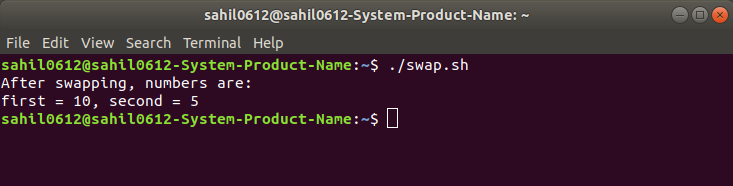# Bash shell script to swap two numbers

Given two numbers and the task is to swap them using the third variable.

Examples:

```Input: first = 2, second = 4
Output: first = 4, second = 2

Input: first = 5, second = 7
Output: first = 7, second = 5
```

Approach:

1. Store the value of the first number into a temp variable.
2. Store the value of the second number in the first number.
3. Store the value of temp into the second variable.

 `# !/bin/bash ` `  `  `# Program to swap two numbers ` `  `  `# Static input of the ` `# number ` `first=5 ` `second=10 ` `  `  `temp=\$first ` `first=\$second ` `second=\$temp ` ` `  `echo` `"After swapping, numbers are:"` `echo` `"first = \$first, second = \$second"`

Output:

```After swapping, numbers are:
first = 10, second = 5
```

How to execute the bash files?

1. Write the bash code into a file and save that file with .sh extension i.e. filename.sh
2. Open terminal and execute the file using below command:
```./filename.sh
```My Personal Notes arrow_drop_upCheck out this Author's contributed articles.

If you like GeeksforGeeks and would like to contribute, you can also write an article using contribute.geeksforgeeks.org or mail your article to contribute@geeksforgeeks.org. See your article appearing on the GeeksforGeeks main page and help other Geeks.

Please Improve this article if you find anything incorrect by clicking on the "Improve Article" button below.

Article Tags :

Be the First to upvote.

Please write to us at contribute@geeksforgeeks.org to report any issue with the above content.